SLVAFJ7 March   2023

This fifth installment of the topology application brief series introduces the inverting buck-boost converter and Ćuk converter. Both topologies allow generation of a negative output voltage from a positive input voltage.

## Inverting Buck-Boost Converters

The inverting buck-boost topology can step up and step down the input voltage while the output voltage is negative. The energy transfers from the input to the output when switch Q1 is not conducting. Figure 1-1 shows the schematic of a nonsynchronous inverting buck-boost converter.

Figure 1-1 Nonsynchronous Inverting Buck-Boost Converter Schematic

Equation 1 calculates the duty cycle in continuous conduction mode (CCM) as:

Equation 1. $\mathrm{D}=\frac{{-\mathrm{V}}_{\mathrm{O}\mathrm{U}\mathrm{T}}+{\mathrm{V}}_{f}}{{-\mathrm{V}}_{\mathrm{O}\mathrm{U}\mathrm{T}}+{\mathrm{V}}_{f}+{\mathrm{V}}_{\mathrm{I}\mathrm{N}}}$

Equation 2 calculates the maximum metal-oxide semiconductor field-effect transistor (MOSFET) stress as:

Equation 2. ${\mathrm{V}}_{\mathrm{Q}1}={{\mathrm{V}}_{\mathrm{I}\mathrm{N}}+{\mathrm{V}}_{f}-\mathrm{V}}_{\mathrm{O}\mathrm{U}\mathrm{T}}$

Equation 3 gives the maximum diode stress as:

Equation 3. ${\mathrm{V}}_{\mathrm{D}1}={{\mathrm{V}}_{\mathrm{I}\mathrm{N}}-\mathrm{V}}_{\mathrm{O}\mathrm{U}\mathrm{T}}$

where

• VIN is the input voltage
• VOUT is the output voltage
• Vƒ is the diode forward
• The value for VOUT needs to be negative for all three equations.

Since there is no inductor-capacitor (LC) filter pointing to the input or output of the inverting buck-boost converter, this topology has pulsed currents at both converter ends, leading to rather high-voltage ripple. For electromagnetic interference (EMI) compliance, additional input filtering might be necessary. If the converter needs to supply a very sensitive load, a second-stage filter at the output might not provide enough damping of the output voltage ripple. In such cases a Ćuk converter is recommended instead.

An inverting buck-boost converter can be built by using a buck controller or converter since a P-channel MOSFET or high-side MOSFET driver is needed. However, the ground terminals of the controller or converter integrated circuit (IC) need to be connected to the negative output voltage. The IC is then regulating the ground signal versus the negative output voltage.

The right half-plane zero (RHPZ) is the limiting factor for the achievable regulation bandwidth of the inverting buck-boost converter. The maximum bandwidth is roughly one-fifth the RHPZ frequency. Equation 4 estimates the single RHPZ frequency of the transfer function of the inverting buck-boost converter:

Equation 4. ${f}_{\mathrm{R}\mathrm{H}\mathrm{P}\mathrm{Z}}=\frac{{\mathrm{V}}_{\mathrm{O}\mathrm{U}\mathrm{T}}×{\left(1-\mathrm{D}\right)}^{2}}{2×\mathrm{\pi }×{\mathrm{D}×\mathrm{L}}_{1}×{\mathrm{I}}_{\mathrm{O}\mathrm{U}\mathrm{T}}}$

where

• VOUT is the output voltage
• D is the duty cycle
• IOUT is the output current
• L1 is the inductance of inductor L1.
• The values for both VOUT and IOUT need to be negative.

Figure 1-2 through Figure 1-7 show voltage and current waveforms in CCM for the FET Q1, inductor L1, and diode D1 in a nonsynchronous inverting buck-boost converter.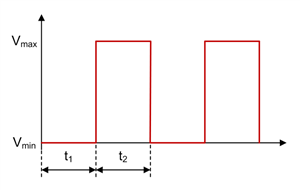Figure 1-2 Inverting Buck-Boost FET Q1 Voltage Waveform in CCMFigure 1-4 Inverting Buck-Boost Inductor L1 Voltage Waveform in CCM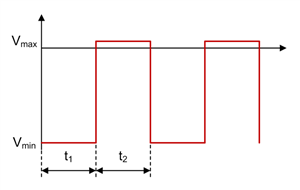Figure 1-6 Inverting Buck-Boost Diode D1 Voltage Waveform in CCM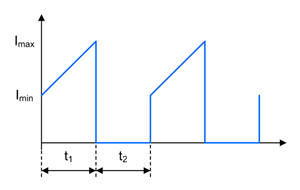Figure 1-3 Inverting Buck-Boost FET Q1 Current Waveform in CCM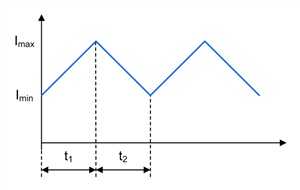Figure 1-5 Inverting Buck-Boost Inductor L1 Current Waveform in CCMFigure 1-7 Inverting Buck-Boost Diode D1 Current Waveform in CCM

## Ćuk Converters

The Ćuk topology can step up and step down the input voltage while the output voltage is negative. The energy transfers from the input to the output when switch Q1 is not conducting. Figure 1-8 shows the schematic of a nonsynchronous Ćuk converter.

Figure 1-8 Nonsynchronous Ćuk Converter Schematic

Equation 5 calculates the duty cycle in CCM as:

Equation 5. $\mathrm{D}=\frac{{-\mathrm{V}}_{\mathrm{O}\mathrm{U}\mathrm{T}}+{\mathrm{V}}_{f}}{{-\mathrm{V}}_{\mathrm{O}\mathrm{U}\mathrm{T}}+{\mathrm{V}}_{f}+{\mathrm{V}}_{\mathrm{I}\mathrm{N}}}$

Equation 6 calculates the maximum MOSFET stress as:

Equation 6. ${\mathrm{V}}_{\mathrm{Q}1}={{\mathrm{V}}_{\mathrm{I}\mathrm{N}}-\mathrm{V}}_{\mathrm{O}\mathrm{U}\mathrm{T}}{+\mathrm{V}}_{f}+\frac{{\mathrm{V}}_{\mathrm{C}\mathrm{1},\mathrm{r}\mathrm{i}\mathrm{p}\mathrm{p}\mathrm{l}\mathrm{e}}}{2}$

Equation 7 gives the maximum diode stress as:

Equation 7. ${\mathrm{V}}_{\mathrm{D}1}={{\mathrm{V}}_{\mathrm{I}\mathrm{N}}-\mathrm{V}}_{\mathrm{O}\mathrm{U}\mathrm{T}}+\frac{{\mathrm{V}}_{\mathrm{C}\mathrm{1},\mathrm{r}\mathrm{i}\mathrm{p}\mathrm{p}\mathrm{l}\mathrm{e}}}{2}$

where

• VIN is the input voltage
• VOUT is the output voltage
• Vf is the diode forward voltage
• VC1,ripple is the voltage ripple across the coupling capacitor C1

The value for VOUT needs to be negative for these three equations.

The LC filter L2 / Co in a Ćuk converter is pointing to the output. As a result, the output ripple is quite small, because the output current is continuous. When viewing the input, there is another LC filter with L1 / Ci. Thus the input current is continuous as well, which results in very small input ripple, too. Thus the Ćuk converter is an excellent fit for applications that require a negative output voltage while being very sensitive at both the input and output, such as power supplies for telecommunications.

A Ćuk converter can easily be built using a boost controller, because MOSFET Q1 needs to be driven on the low side. The boost converter or controller IC typically only accepts a positive feedback voltage at the feedback pin. The negative output voltage can be translated into a positive voltage signal by using a simple inverting operational amplifier circuit.

The Ćuk converter has an RHPZ as well. The power stage cannot immediately react to changes at the output, because the energy transfers to the output when switch Q1 is off. The maximum achievable crossover frequency is again one-fifth the RHPZ frequency. Note that a Ćuk converter has more than one RHPZ. Equation 8 estimates one of the RHPZs of the Ćuk converter as:

Equation 8. ${f}_{\mathrm{R}\mathrm{H}\mathrm{P}\mathrm{Z}}=\frac{1}{2×\mathrm{\pi }}×\sqrt{\frac{1-\mathrm{D}}{{\mathrm{L}}_{1}{×\mathrm{C}}_{1}}}$

where

• D is the duty cycle
• L1 is the inductance of inductor L1
• C1 is the capacitance of coupling capacitor C1

Figure 1-9 through Figure 1-18 show voltage and current waveforms in CCM for the FET Q1, inductor L1, coupling capacitor C1, diode D1, and inductor L2 in a nonsynchronous Ćuk converter.Figure 1-9 Ćuk FET Q1 Voltage Waveform in CCM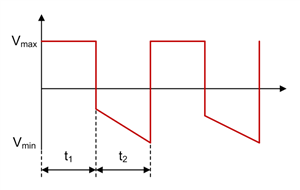Figure 1-11 Ćuk Inductor L1 Voltage Waveform in CCM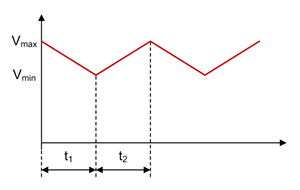Figure 1-13 Ćuk Coupling Capacitor C1 Voltage Waveform in CCM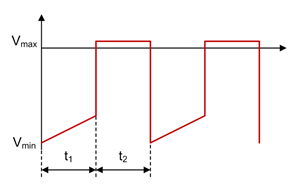Figure 1-15 Ćuk Diode D1 Voltage Waveform in CCM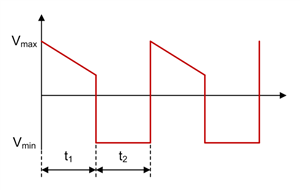Figure 1-17 Ćuk Inductor L2 Voltage Waveform in CCM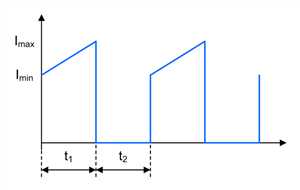Figure 1-10 Ćuk FET Q1 Current Waveform in CCM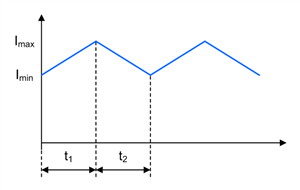Figure 1-12 Ćuk Inductor L1 Current Waveform in CCM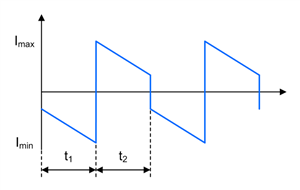Figure 1-14 Ćuk Coupling Capacitor C1 Current Waveform in CCM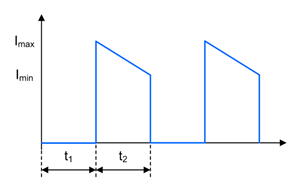Figure 1-16 Ćuk Diode D1 Current Waveform in CCM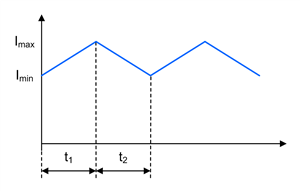Figure 1-18 Ćuk Inductor L2 Current Waveform in CCM

Much like for a single-ended primary inductance converter (SEPIC) and Zeta converter, it can also make sense to use coupled inductors for a Ćuk converter instead of two separate inductors. Using coupled inductors offers two advantages: The first benefit is that only half the inductance compared to a two-inductor design is required for similar current ripple because coupling the windings leads to ripple cancellation. The second benefit is that the resonance in the transfer function caused by the two inductors and the coupling capacitor can be removed. This resonance usually needs to be damped by a resistor-capacitor (RC) network in parallel with coupling capacitor C1.

One drawback to using coupled inductors is that the same inductance value for both inductors must be used. Another limitation of coupled inductors is typically their current rating. For applications with high-output currents, there might be no other option than to use single inductors.

Configure both inverting buck-boost and Ćuk converters as a converter with synchronous rectification if the application requires output currents greater than 3 A. If implementing synchronous rectification for a Ćuk converter, AC-couple the high-side gate-drive signal, because many controllers are required to connect them to the switch node. The Ćuk converter has two switch nodes, so take care to avoid negative voltage rating violations on the SW pin.